# Neo4j - CQL 查询语句的简介【纯个人总结】

【摘要】最近在做商品关联性分析（商品推荐），考虑到若使用关系型数据库会比较啰嗦麻烦，所以采用图数据库，这里就需要去学习图数据库的一些知识，本文总结了一款主流图数据库 neo4j 的查询方法，就像 MySQL 数据库的查询 SQL 一样，主要是为了供后期实际开发时候，个人快速搜索参考使用。

## （一）创建节点

(1)通过一条语句创建一个节点

1. `# return a 表示创建之后并返回节点 a`
2. `# return 语句可省略`
3. `# 创建一个标签为 Student 且无属性的节点，创建之后，可以新增、修改属性`
4. `create(a:Student) return a`
5. `# 创建一个标签为 Student 并设置它的属性 stu_no = '10003',age = 22,name = '张三'`
6. `create(a:Student{stu_no:'10003',age:22,sex:'男',name:'张三'}) return a `

(2)通过一条语句创建多个节点

1. `# 创建多个节点时，使用逗号 ',' 连接节点`
2. `# return a,b,c 表示创建之后并返回节点 a,b,c`
3. `# return 语句可省略`
4. `create (a:Student{stu_no:'10004',age:22,sex:'男',name:'李四'}),`
5. `(b:Student{stu_no:'10005',age:23,sex:'男',name:'王五'}),`
6. `(c:Student{stu_no:'10006',age:22,sex:'男',name:'赵六'}) `
7. `return a,b,c`

## （二）查询节点

(1)查询所有节点、所有节点之间的关系：

1. `# 查询所有数据（节点 + 关系）`
2. `match(n) return n`
3. `match data = ()-[]->() return data`
4. `# 查询标签为 Student 的所有节点`
5. `match(n:Student) return n`
6. `# 查询标签为 Student 的属性: name,age,sex `
7. `match(n:Student) return n.name,n.age,n.sex `

(2)查询指定的节点

1. `# where、and、or 、in、() 语句的使用`
2. `match(n) where n.name = '张三' and n.age = 22 return n`
3. `match(n) where n.stu_no = '10003' or n.name = '李四' return n`
4. `match(n) where n.name in ['张三','李四'] return n`
5. `match(n) where n.name = '张三' and (n.stu_no = '10003' or n.age = 20) return n`

(3)模糊查询

1. `# 使用 =~、.*`
2. `# 返回 name 中带有 "张" 的节点`
3. `match(n) where n.name =~ '.*张.*' return n `
4. `# 返回 name 开头为 "李" 的节点`
5. `match(n) where n.name =~ '李.*' return n`

(4) limit,skip 语句

1. `# 使用 limit 限制查询返回的行数`
2. `match(n) return n limit 10 `
3. `# 使用 skip n 跳过前 n 行`
4. `match(n) return n skip 2 limit 10`

(5) order by 语句

1. `# desc：降序/asc：升序`
2. `match(n) return n.name,n.age,n.sex order by n.age desc`

(6) null 关键词

1. `# is,is not 关键词`
2. `match(n) where n.sex is not null return n`

(7)聚合函数 count()/max()/min()/avg() 等关键词

1. `match(n:Student) return count(n)`
2. `match(n:Student) return max(n.age)`
3. `match(n:Student) return min(n.age)`
4. `match(n:Student) return avg(n.age)`
5. `# select *,min(age) 方式用法`
6. `match(n:Student) return n,min(n.age)`

(8)将结果赋值给变量后返回变量

1. `match data = (a:Student) where a.name = '张三' return data`

## （三）创建节点之间的关系

(1)创建已存在节点之间的关系

1. `# 创建节点的属性 name 值为 "张三"、"李四" 之间的关系`
2. `# return r ：返回关系`
3. `# 备注：关系是可以重复的，即重复执行会生成多个关系，且互不影响`
4. `match (a:Student),(b:Student)`
5. `where a.name = '张三' and b.name = '李四'`
6. `create (a)-[r:friendship{score:1}]->(b)`
7. `return r`
8. `match (a:Student),(b:Student)`
9. `where a.name = '张三' and b.name = '王五'`
10. `create (a)-[r:friendship{score:3}]->(b)`
11. `return r`
12. `match (a:Student),(b:Student)`
13. `where a.name = '张三' and b.name = '赵六'`
14. `create (a)-[r:friendship{score:2}]->(b)`
15. `return r`

(2)创建节点的同时创建节点之间的关系

1. `# 在同一条语句中创建新节点的同时也创建了节点之间的关系`
2. `create (a:Student{stu_no:'10007',age:21,sex:'男',name:'孙七'})-[r:friendship{score:1}]->(b:Student{stu_no:'10008',age:22,sex:'男',name:'周八'}) `
3. `return a,b,r`

(3)在同一个执行体内执行创建节点并创建节点之间的关系

1. `# 下面三条语句必须在一个执行体内完成，否则在创建关系时括号 () 中的节点会被认为是新的节点`
2. `create(a:Student{stu_no:'10009',age:22,sex:'男',name:'吴九'})`
3. `create(b:Student{stu_no:'10010',age:23,sex:'男',name:'郑十'})`
4. `create(a)-[r:friendship{score:8}]->(b) return a,b,r`
5. `补充数据：`
6. `match(a:Student),(b:Student),(c:Student) `
7. `where a.name = '张三' and b.name = '吴九' and c.name = '孙七' `
8. `create (a)-[re1:friendship{score:5}]->(b),(a)-[re2:friendship{score:8}]->(c) `
9. `return a,b,c,re1,re2`

1. `match(n:Student) return n`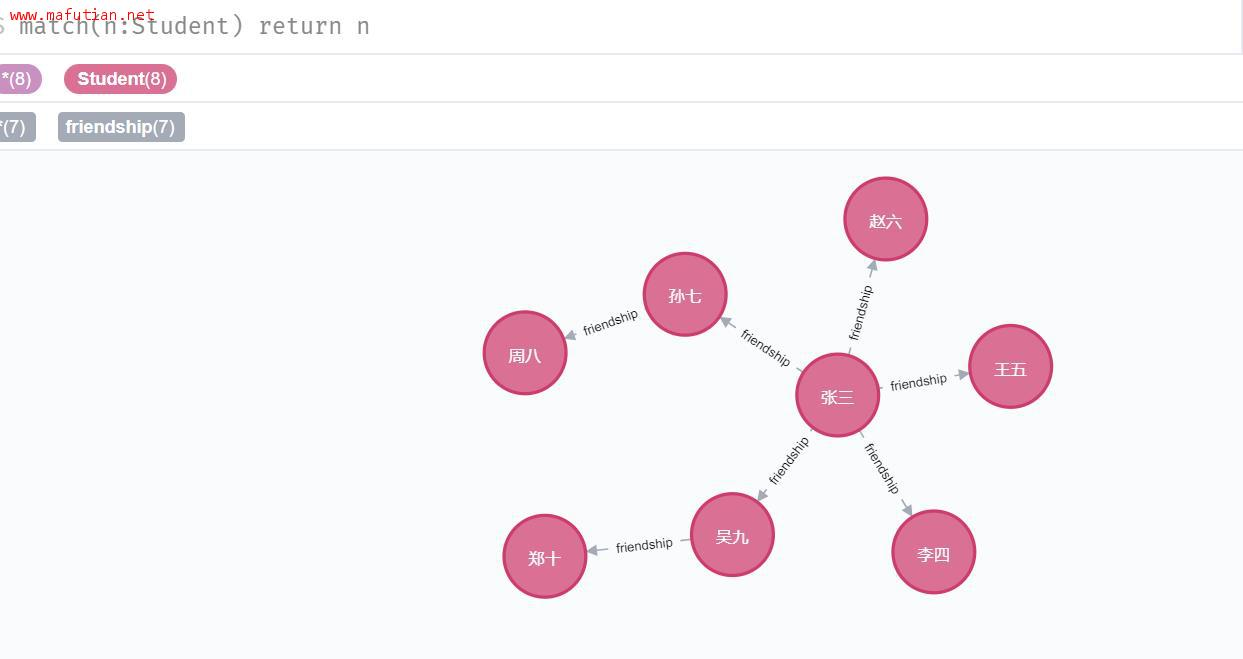## （四）查询节点与节点之间的关系

(1)查询所有节点之间的关系

1. `# type(r) 表示关系名`
2. `# 以下几种方式都可以返回节点的关系`
3. `match()-[r]-() return r`
4. `match()-[r]->() return r`
5. `match()<-[r]-() return r`
6. `match()<-[r]->() return r,type(r)`
7. `# 按条件查询节点之间的关系`
8. `match()-[r]-() where r.score = 2 return r,type(r)`
9. `# 查询所有符合条件的关系的节点及关系`
10. `match(a)-[r]->(b) where r.score = 2 return a,b,r`
11. `# 查询某个节点的所有关系`
12. `match(a:Student)-[r:friendship]->() return r,type(r)`

## （五）删除节点、节点之间的关系

1. `# 删除所有节点、所有节点之间的关系`
2. `match(n) detach delete n`
3. `# 按条件删除两个节点之间的关系`
4. `match (a:Student)-[r]->(b:Student) `
5. `where a.name = '张三' and b.name = '王五' `
6. `delete r`

## （六）添加/修改/删除节点的属性

1. `# 使用 set 语句进行添加、修改，如果 set 属性不存在则创建，存在则修改`
2. `match(n) where n.name = '张三' set n.age = 30 return n`
3. `# 新增属性 address，并设值为 "北京市朝阳区双井街道双花园南里"`
4. `match(n) where n.name = '张三' set n.address = '北京市朝阳区双井街道双花园南里' return n `
5. `# 使用 remove 语句进行属性的删除`
6. `match(n) where n.name = '张三' remove n.address return n`

## （七）关联查询（深度查询，查询子级的子级）

1. `# 使用 with 语句`
2. `match(a:Student)-[re1]->(b:Student) `
3. `where a.name = '张三' `
4. `with a,re1,b match(b:Student)-[re2]->(c:Student) `
5. `return a,b,c,re1,re2`
6. `# 或者直接拼接方式连接`
7. `match(a:Student)-[re1]->(b:Student)-[re2]->(c:Student) `
8. `return a,b,c,re1,re2`

## （八）综合应用

(1)查询节点为 "张三" 的所有朋友，并按照好友关系度 score 从高到低排序，返回前三条数据

1. `match(n:Student)-[r:friendship]->(m) `
2. `where n.name = '张三' `
3. `return n,r,m `
4. `order by r.score desc `
5. `limit 3`

(2)查询节点为 "张三" 的好友的好友关系 friendship 的 score 大于 5 的好友名称

1. `match(a:Student)-[re1]->(b:Student) `
2. `where a.name = '张三' `
3. `with a,b,re1 match(b:Student)-[re2]->(c:Student) `
4. `where re2.score > 5 `
5. `return c.name`

(1)create 可以用来创建节点、节点之间的关系

(2)match 用来查询节点，where 是 match 查询时的过滤条件

(3)match 后可以接删除 delete 语句

(4)删除节点的时候，需要先删与该节点有关的关系，再删节点

(5)以上总结的并不是很全面，只是将可能会经常用到的查询写出来了，入个门，具体的掌握还需要通过实际的操作来加强，更多详情可以参考网址：https://www.w3cschool.cn/neo4j/

0

0

 评论审核未开启 回复后邮件通知我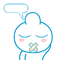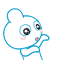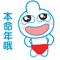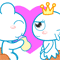• 浏览最多
• 评论最多